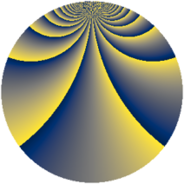# Properties

 Label 2280.2.bpLevel $2280$ Weight $2$ Character orbit 2280.bp Rep. character $\chi_{2280}(77,\cdot)$ Character field $\Q(\zeta_{4})$ Dimension $864$ Sturm bound $960$

# Related objects

## Defining parameters

 Level: $$N$$ $$=$$ $$2280 = 2^{3} \cdot 3 \cdot 5 \cdot 19$$ Weight: $$k$$ $$=$$ $$2$$ Character orbit: $$[\chi]$$ $$=$$ 2280.bp (of order $$4$$ and degree $$2$$) Character conductor: $$\operatorname{cond}(\chi)$$ $$=$$ $$120$$ Character field: $$\Q(i)$$ Sturm bound: $$960$$

## Dimensions

The following table gives the dimensions of various subspaces of $$M_{2}(2280, [\chi])$$.

Total New Old
Modular forms 976 864 112
Cusp forms 944 864 80
Eisenstein series 32 0 32

## Trace form

 $$864q + O(q^{10})$$ $$864q + 16q^{10} + 16q^{22} - 32q^{28} + 40q^{30} - 16q^{36} - 56q^{40} + 40q^{42} - 52q^{48} - 56q^{52} - 32q^{58} - 100q^{60} - 72q^{66} - 40q^{70} - 100q^{72} - 128q^{78} + 32q^{81} - 24q^{82} + 112q^{88} - 136q^{90} + 40q^{96} - 32q^{97} + O(q^{100})$$

## Decomposition of $$S_{2}^{\mathrm{new}}(2280, [\chi])$$ into newform subspaces

The newforms in this space have not yet been added to the LMFDB.

## Decomposition of $$S_{2}^{\mathrm{old}}(2280, [\chi])$$ into lower level spaces

$$S_{2}^{\mathrm{old}}(2280, [\chi]) \cong$$ $$S_{2}^{\mathrm{new}}(120, [\chi])$$$$^{\oplus 2}$$